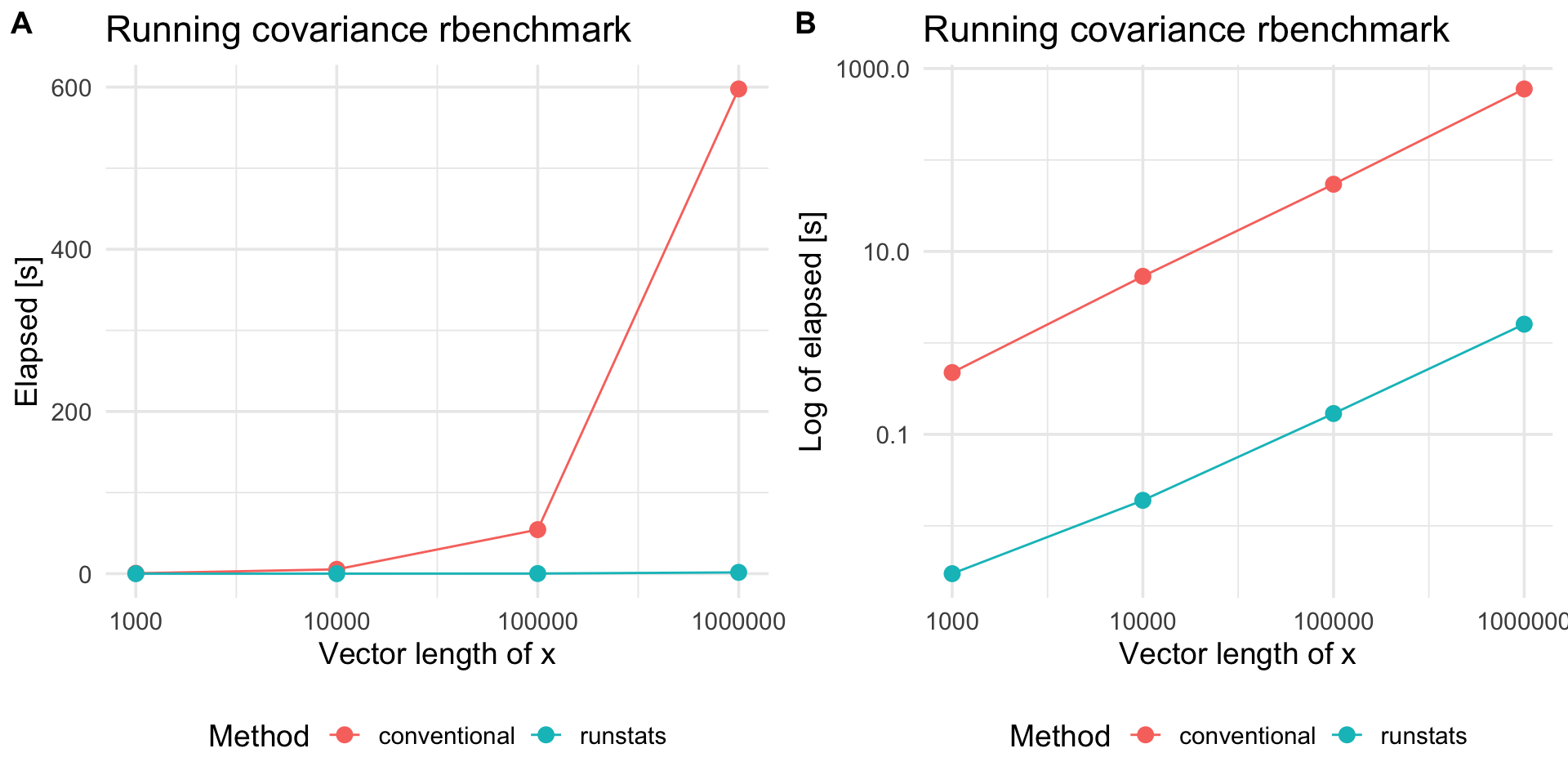Package runstats provides methods for fast computation of running sample statistics for time series. The methods utilize Convolution Theorem to compute convolutions via Fast Fourier Transform (FFT). Implemented running statistics include:

1. mean,
2. standard deviation,
3. variance,
4. covariance,
5. correlation,
6. euclidean distance.

### Website

Package website is located here.

### Installation

# devtools::install_github("martakarass/runstats")
install.packages("runstats")

### Usage

library(runstats)

## Example: running correlation
x0 <- sin(seq(0, 2 * pi * 5, length.out = 1000))
x  <- x0 + rnorm(1000, sd = 0.1)
pattern <- x0[1:100]
out1 <- RunningCor(x, pattern)
out2 <- RunningCor(x, pattern, circular = TRUE)

## Example: running mean
x <- cumsum(rnorm(1000))
out1 <- RunningMean(x, W = 100)
out2 <- RunningMean(x, W = 100, circular = TRUE)

### Running statistics

To better explain the details of running statistics, package’s function runstats.demo(func.name) allows to visualize how the output of each running statistics method is generated. To run the demo, use func.name being one of the methods’ names:

1. "RunningMean",
2. "RunningSd",
3. "RunningVar",
4. "RunningCov",
5. "RunningCor",
6. "RunningL2Norm".
## Example: demo for running correlation method
runstats.demo("RunningCor")## Example: demo for running mean method
runstats.demo("RunningMean")### Performance

We use rbenchmark to measure elapsed time of RunningCov execution, for different lengths of time-series x and fixed length of the shorter pattern y.

library(rbenchmark)

set.seed (20181010)
x.N.seq <- 10^(3:7)
x.list  <- lapply(x.N.seq, function(N) runif(N))
y <- runif(100)

## Benchmark execution time of RunningCov
out.df <- data.frame()
for (x.tmp in x.list){
out.df.tmp <- benchmark("runstats" = runstats::RunningCov(x.tmp, y),
replications = 10,
columns = c("test", "replications", "elapsed",
"relative", "user.self", "sys.self"))
out.df.tmp$x_length <- length(x.tmp) out.df.tmp$pattern_length <- length(y)
out.df <- rbind(out.df, out.df.tmp)
}
knitr::kable(out.df)
test replications elapsed relative user.self sys.self x_length pattern_length
runstats 10 0.005 1 0.004 0.001 1000 100
runstats 10 0.023 1 0.018 0.004 10000 100
runstats 10 0.194 1 0.158 0.037 100000 100
runstats 10 1.791 1 1.656 0.125 1000000 100
runstats 10 20.234 1 17.660 2.514 10000000 100
##### Compare with a conventional method

To compare RunStats performance with “conventional” loop-based way of computing running covariance in R, we use rbenchmark package to measure elapsed time of RunStats::RunningCov and running covariance implemented with sapply loop, for different lengths of time-series x and fixed length of the shorter time-series y.

## Conventional approach
RunningCov.sapply <- function(x, y){
l_x <- length(x)
l_y <- length(y)
sapply(1:(l_x - l_y + 1), function(i){
cov(x[i:(i+l_y-1)], y)
})
}

set.seed (20181010)

out.df2 <- data.frame()
for (x.tmp in x.list[c(1,2,3,4)]){
out.df.tmp <- benchmark("conventional" = RunningCov.sapply(x.tmp, y),
"runstats" = runstats::RunningCov(x.tmp, y),
replications = 10,
columns = c("test", "replications", "elapsed",
"relative", "user.self", "sys.self"))
out.df.tmp\$x_length <- length(x.tmp)
out.df2 <- rbind(out.df2, out.df.tmp)
}

Benchmark results

library(ggplot2)

plt1 <-
ggplot(out.df2, aes(x = x_length, y = elapsed, color = test)) +
geom_line() + geom_point(size = 3) + scale_x_log10() +
theme_minimal(base_size = 14) +
labs(x = "Vector length of x",
y = "Elapsed [s]", color = "Method",
title = "Running covariance rbenchmark") +
theme(legend.position = "bottom")
plt2 <-
plt1 +
scale_y_log10() +
labs(y = "Log of elapsed [s]")

cowplot::plot_grid(plt1, plt2, nrow = 1, labels = c('A', 'B'))Platform information

sessioninfo::platform_info()
#>  setting  value
#>  version  R version 3.5.2 (2018-12-20)
#>  os       macOS Mojave 10.14.2
#>  system   x86_64, darwin15.6.0
#>  ui       X11
#>  language (EN)
#>  collate  en_US.UTF-8
#>  ctype    en_US.UTF-8
#>  tz       America/New_York
#>  date     2019-11-14